## Excel GROWTH Function

The GROWTH function returns the predicted exponential GROWTH based on a given set of data. By using the existing x-values and y-values, the GROWTH function calculates the predicted y-values for a series of new x-values. In financial terms, companies can use the GROWTH function to predict the revenues of the coming years.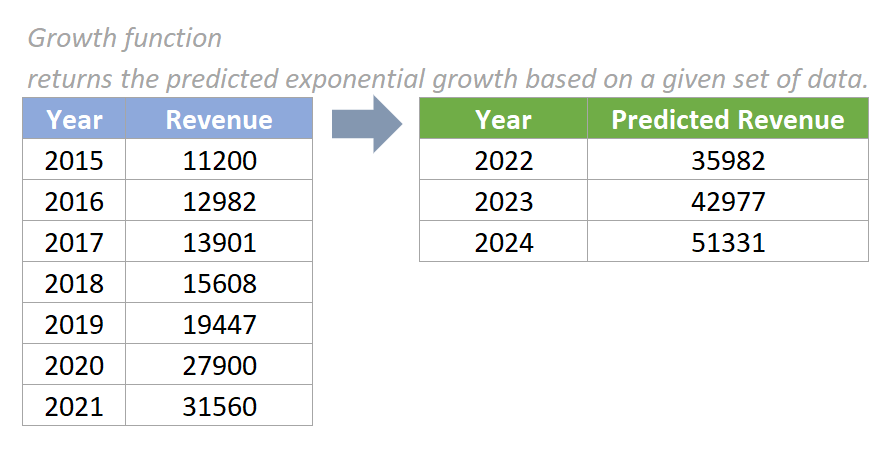#### Syntax

=GROWTH(known_y’s,[known_x’s],[new_x’s],[const])

#### Arguments

• Known_y’s (required): A set of known y-values used to predict the exponential GROWTH.
• Known_x’s (optional): A set of known x-values. If provided, the data range of known_x’s should have the same length as the data range of known_y’s. If omitted, it uses{1,2,3,...} as the known_y’s parameter.
• New_x’s (optional): A set of new x-values, for which you want to get corresponding new y-values. If omitted, new_x’s is assumed to be the same as known_x’s.
• Const (optional): A logical value specifying whether the constant 'b', in the equation y = b * m^x, should be forced to be equal to the value 1. It is either a TRUE or FALSE value.
If const is TRUE or omitted, the constant b is calculated normally.
If const is FALSE, the constant b is set to 1 and the equation becomes y = m^x.

#### Return Value

The GROWTH function returns a numeric value.

#### Function notes

1. We can use the GROWTH function as an array formula by pressing the Ctrl + Shift+ Enter keys. But in Excel for Microsoft 365, new dynamic array functions are introduced, meaning no need to use Ctrl + Shift+ Enter to enter the GROWTH formula as an array formula.
2. The #REF! error value occurs if the array of known_x’s has a different length from the known_y’s array.
3. The #NUM! error value occurs if any value in the array of known_y’s is less than or equal to zero.
4. The #VALUE! error value occurs if any of the values in the supplied known_y’s, known_x’s, or new_x’s arrays are non-numeric.
5. While using the GROWTH function in graphs, we can create a scatter chart of the data without displaying a line and use the exponential option from the graph trendline option.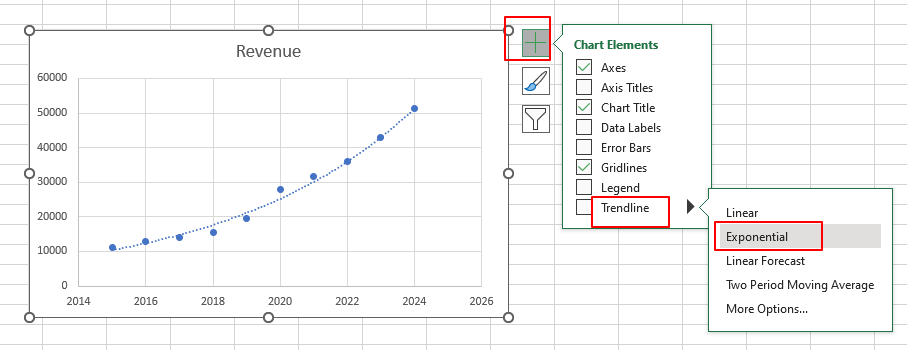#### Example

##### Example One: Basic Usage

As the below screenshot shows, there are the report data of annual revenues between year 2015 to 2021. To predict the revenues of year from 2022 to 2024, please do as follows:

1. Please copy the formula below into cell F5, then press the Enter key to get the result.

=GROWTH(C5:C11,B5:B11,E5)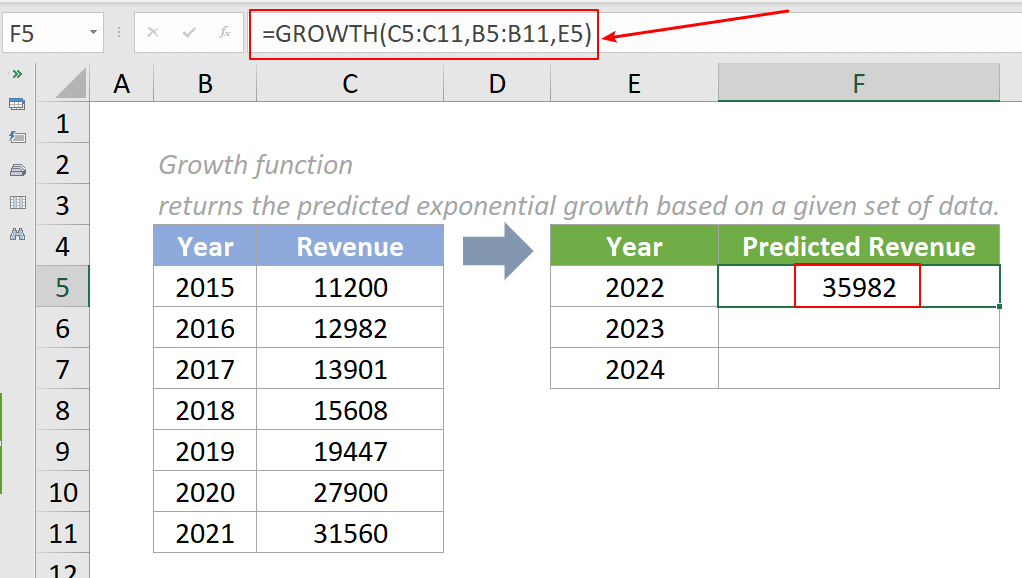2. To get the rest of the results, we need to convert known_y’s and known_x’s arguments to absolute references in the formula.

In the above formula, you need to convert the cell references C5:C11 and B5:B11 to absolute references. Please select the data range of known_y’s C5:C11, then press the F4 key to convert it to an absolute reference. Repeat the same step to convert the data range of known_x’s B5:B11 to an absolute reference. Then you can see the formula displayed as follows:

=GROWTH(\$C\$5:\$C\$11,\$B\$5:\$B\$11,E5)

3. Select this result cell and drag its autofill handle down to get the rest of the results.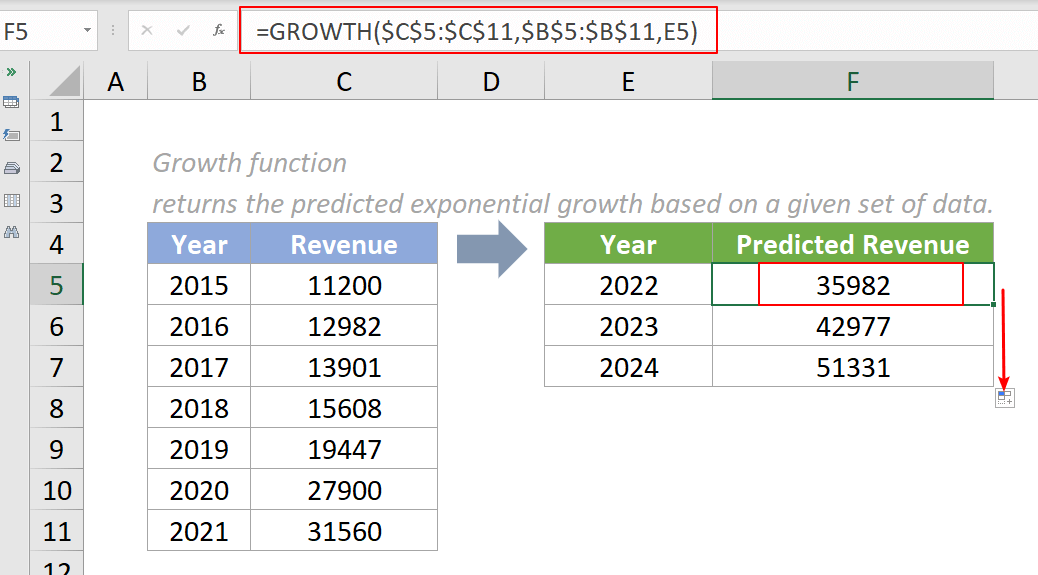##### Example Two: Use as an Array Formula

The GROWTH function can be entered as an array formula. To predict the revenues of year from 2022 to 2024 based on the data in the example one, please do as follows:

1. Select the range where we want the estimated revenues to be returned.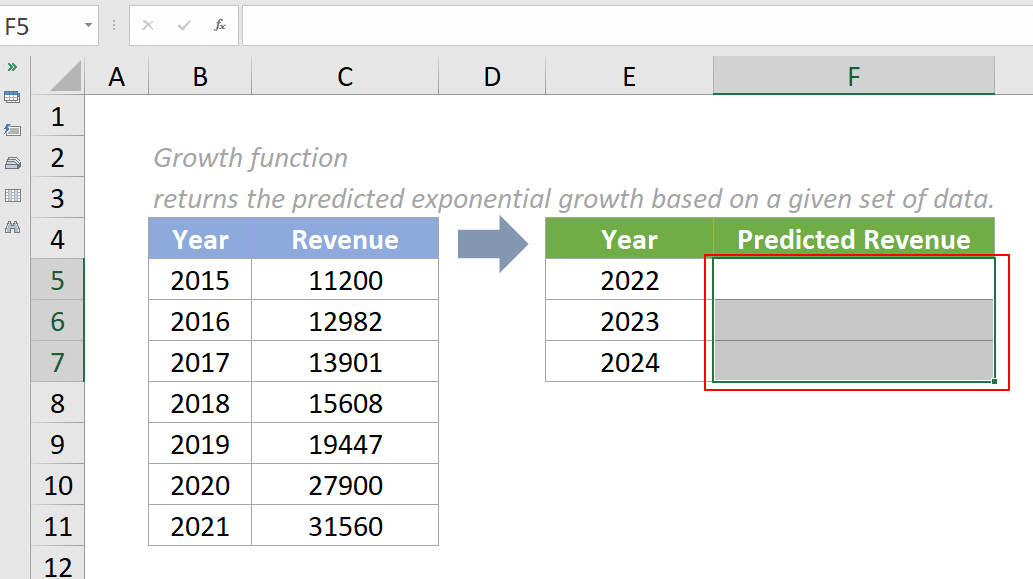2. Please copy the formula below into the Formula Bar , then press CTRL+SHIFT+ENTER to get the results.

=GROWTH(C5:C11,B5:B11,E5:E7)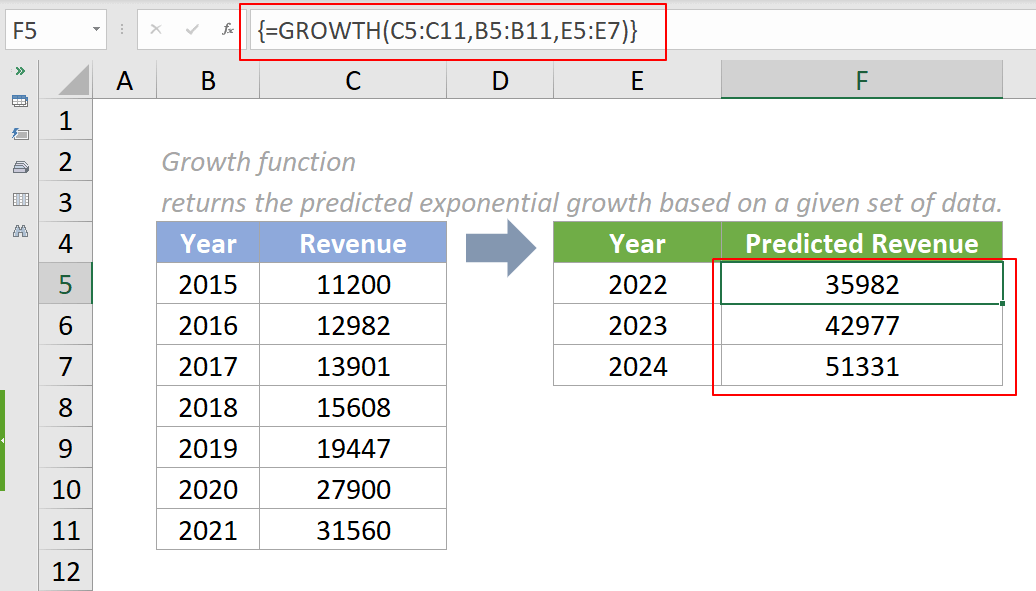Notes:

1. You can see the formula above is encased in curly braces { }, meaning that it was entered as an array formula.
2. Dynamic array formulas are available in Excel 365, Excel 2021, and Excel for the web, meaning no need to press CTRL+SHIFT+ENTER to make the formula dynamic. For users of Excel 2019 and earlier, remember to enter the formula via CTRL+SHIFT+ENTER so the multiple results can be returned in an array.

#### Relative Functions:

• Excel EVEN Function
The EVEN function rounds numbers away from zero to the nearest even integer.

• Excel EXP Function
The EXP function returns the result of the constant e raised to the nth power.